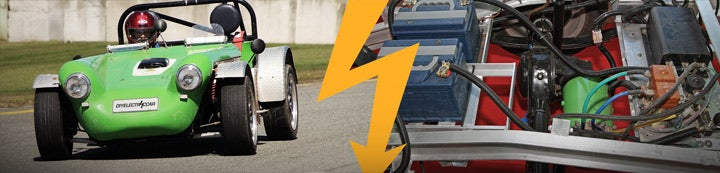# acceleration

1. ### Heavy Mathematical magic - Calculating Acceleration from Torque

Technical Discussion
Okay, so I have a wheel of radius r (Meters), attached to a vehicle of weight M (Kilograms). If I apply a constant torque T (Newton-Meters), how much acceleration do I get? My shaky math comes out to: Inertia I=W*r² Acceleration = T/I (In Radians/sec) Therefore Acceleration = (T/I)*r (In...
2. ### S-10 with a Kelly 650 AMP

EV Performance
I had an S-10 with a Curtis 500 amp. Did 77MPH. I sold it,:(, and bought a better S-10 and have installed a Kelly 650 AMP controller. Problem is, I'm only getting 45mph. Dont have amp meter yet, so I'm not sure what its putting out, but with the old truck, I had a Kelly 500 AMP and it only...